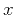Asymptotic pseudounitary stacking operatorsNext: ASYMPTOTIC INVERSION: RECONSTRUCTING THE Up: Asymptotic pseudounitary stacking operators Previous: Introduction

# THEORETICAL DEFINITION OF A STACKING OPERATOR

In practice, integration of discrete data is performed by stacking. In theory, it is convenient to represent a stacking operator in the form of a continuous integral:(1)

Functionis the input of the operator,is the output,is the summation aperture,represents the summation path, andstands for the weighting function. The range of integration (the operator aperture) may also depend onand. Allowingto be a two-dimensional variable, we can use definition (1) to represent an operator applied to three-dimensional data. Throughout this paper, I assume thatandbelong to a one-dimensional space, and thatandhave the same number of dimensions.

The goal of inversion is to reconstruct some functionfor a given, so thatis in some sense close toin equation (1).Asymptotic pseudounitary stacking operatorsNext: ASYMPTOTIC INVERSION: RECONSTRUCTING THE Up: Asymptotic pseudounitary stacking operators Previous: Introduction

2013-03-03